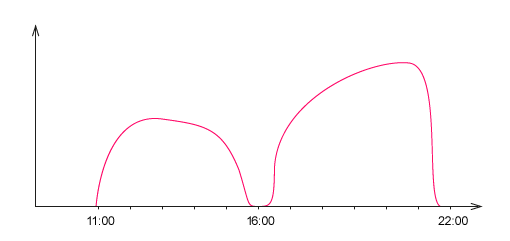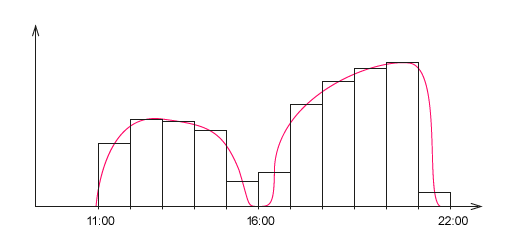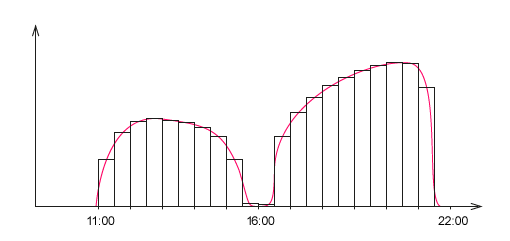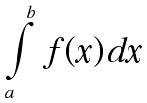# Integral

No, why do I need it?
No, just out of curiosity. Actually, integrals are included even in the school curriculum, but not many people around remember what it is.

Imagine that we have some kind of dependency function on something.For example, you can roughly imagine on a graph the speed of my work depending on the time of day:I measure speed in lines of code per minute, in real life I am a programmer.The amount of work is the speed of my work multiplied by the time of day. That is, if I write 3 lines per minute, I get 180 per hour. If we have such a graph, you can find out how much work I did in a day: this is the area under the graph. But how do I calculate it?
Let's divide the chart into columns of equal width with the value per hour. And the height of these bars will be equal to the work speed in the middle of this hour.The area of each column individually is easy to calculate, you need to multiply its width by height. It turns out that the area of each column is approximately how much work I did for each hour. And if you sum up all the columns, you get an approximate amount of my work for the day.The problem is that the result will be approximate, but we need the exact number. Break the graph into columns by half an hour:You can see in the picture that this is much closer to what we are looking for.In this way, you can reduce the intervals on the chart to infinity, and each time we get closer and closer to the area under the chart. And when the width of the bars will tend to zero, then the sum of their areas will tend to the area under the chart. This is called an integral and is denoted like this:In this formula, f(x) means a function that depends on the value of x, and the letters a and b are the segment on which we want to find the integral.

Why do we need it?
Scientists try to express all physical phenomena as a mathematical formula. Once we have a formula, we can use it to calculate anything further. And the integral is one of the main tools for working with functions.For example, if we have a formula for a circle, we can use the integral to calculate its area. If we have a formula for a ball, we can calculate its volume. With integration, we find energy, work, pressure, mass, electric charge and many other values.

### Why does an engineer need mathematics?

Well, it is clear why - you will answer quickly. - An engineer is nowhere without mathematics! And how long and meticulous is it to know mathematics in order to work successfully in engineering? You will not answer so quickly).

## Where is mathematics used in engineering?

What mathematics is needed to work as an engineer?Will an engineer need to prove theorems or deal with complex numbers in his daily work? Hardly...
But vector analysis can be very useful. As a rule, forces acting in elements of building and engineering constructions are represented by vectors. It is useful for the future engineer to learn how to fold them, to understand the difference between scalar value and vector value.By the way, in the course of theoretical mechanics these questions are given several lessons in the section of static:

Class 1. Vectors. Folding and subtraction of vectors. Projection and vector components. Vector length and direction. Single direction vector. Force expression in vector form.
Class 2. Scalar work of vectors. An example of force component calculation in the rods of a spatial system. Vector product of vectors. Rule of the right hand At what level should differentials and integrals be known? It's enough to devote a few days to these questions, not more, - says Bogdan.It is not necessary to go to the university and study these topics for six months.

An engineer should understand what the maximum and minimum functions are.Matrix calculations also play an important role in engineering activities. Actually, the finite element method and programs related to simulations are based on matrix calculations. You need to understand how matrices can be folded, transformed, etc.But whether it is necessary all in such serious volume to the usual engineer who uses in the work the settlement program? A controversial question...

A specialist who works closely with calculations and often has to analyze the resulting model may have to dig deeper as the results produced by an engineering program often require further analysis and processing.An ordinary design engineer does not need this knowledge much.Probability theory finds its application in work with standards (Eurocodes, for example).Statistics may not be necessary for everyone.

History of Cricket in England https://letswiner.co.uk/history-of-cricket-in-england/

And with such a handy tool at hand as Excel spreadsheets, statistical calculations are easy to do.